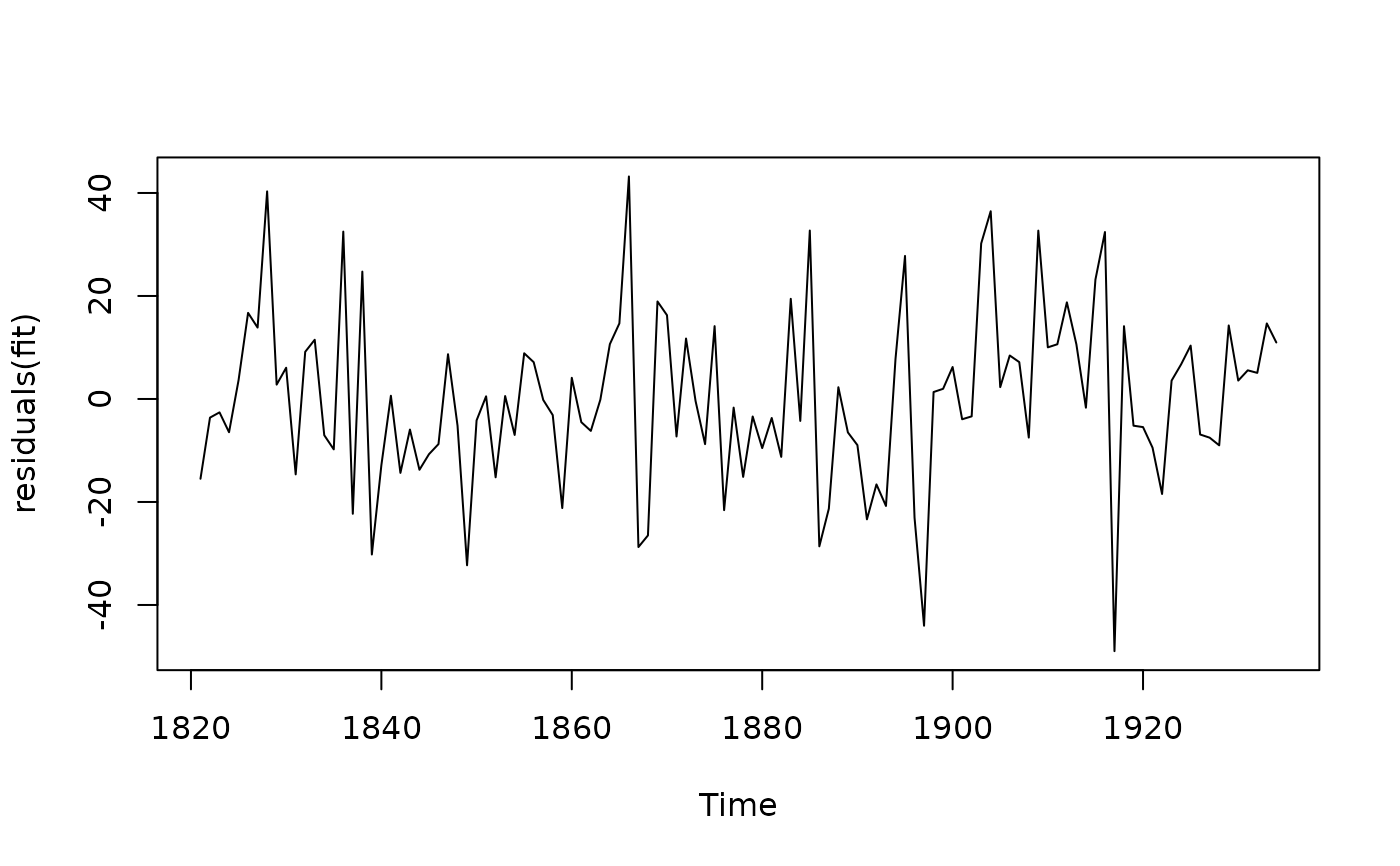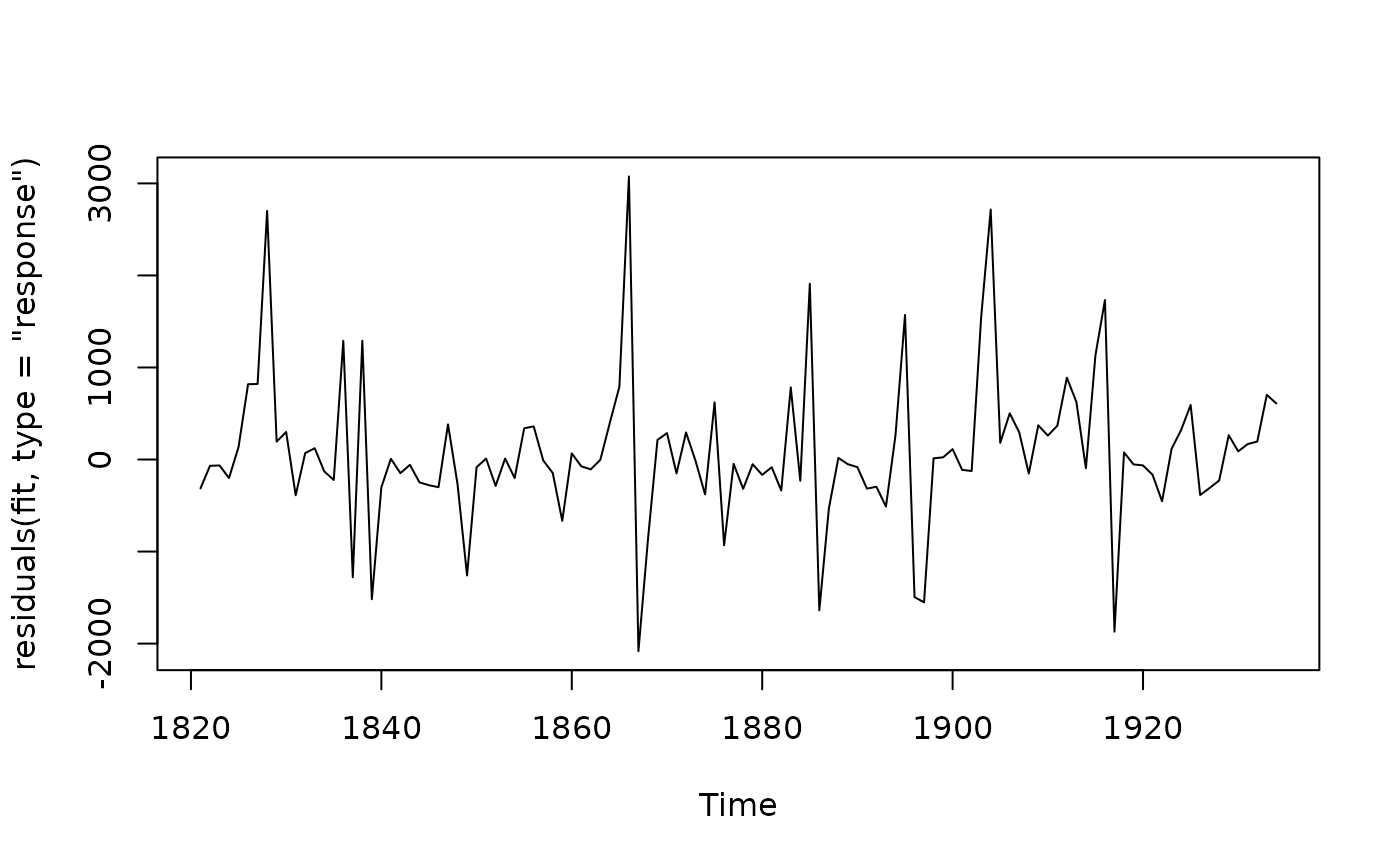Returns time series of residuals from a fitted model.

# S3 method for forecast
residuals(object, type = c("innovation", "response"), ...)

# S3 method for ar
residuals(object, type = c("innovation", "response"), ...)

# S3 method for Arima
residuals(object, type = c("innovation", "response", "regression"), h = 1, ...)

# S3 method for bats
residuals(object, type = c("innovation", "response"), h = 1, ...)

# S3 method for tbats
residuals(object, type = c("innovation", "response"), h = 1, ...)

# S3 method for ets
residuals(object, type = c("innovation", "response"), h = 1, ...)

# S3 method for ARFIMA
residuals(object, type = c("innovation", "response"), ...)

# S3 method for nnetar
residuals(object, type = c("innovation", "response"), h = 1, ...)

# S3 method for stlm
residuals(object, type = c("innovation", "response"), ...)

# S3 method for tslm
residuals(object, type = c("innovation", "response", "deviance"), ...)

## Arguments

object An object containing a time series model of class ar, Arima, bats, ets, arfima, nnetar or stlm. If object is of class forecast, then the function will return object\$residuals if it exists, otherwise it returns the differences between the observations and their fitted values. Type of residual. Other arguments not used. If type='response', then the fitted values are computed for h-step forecasts.

## Value

A ts object.

## Details

Innovation residuals correspond to the white noise process that drives the evolution of the time series model. Response residuals are the difference between the observations and the fitted values (equivalent to h-step forecasts). For functions with no h argument, h=1. For homoscedastic models, the innovation residuals and the response residuals for h=1 are identical. Regression residuals are available for regression models with ARIMA errors, and are equal to the original data minus the effect of the regression variables. If there are no regression variables, the errors will be identical to the original series (possibly adjusted to have zero mean). arima.errors is a deprecated function which is identical to residuals.Arima(object, type="regression"). For nnetar objects, when type="innovations" and lambda is used, a matrix of time-series consisting of the residuals from each of the fitted neural networks is returned.

fitted.Arima, checkresiduals.

Rob J Hyndman

## Examples

fit <- Arima(lynx,order=c(4,0,0), lambda=0.5)

plot(residuals(fit))plot(residuals(fit, type='response'))